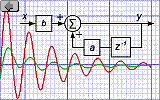Signal Processing

# An IIR Filter in an 8-bit Microcontroller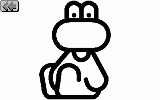www.e-rokodelnica.si

An IIR (Infinite Impulse Response) filters are a very useful tool for attenuating noise in signals that are processed inside microcontrollers, DSP's, FPGA's etc. Architecture and instructions of DSP's (Digital Signal Processors) are designed to implement IIR filters efficiently. 16- and 32- bit processors usually lack some functionality of DSP's. Still, an implementation of IIR filters is quite easy. On the other hand, 8-bit processors are not quite suitable for the job. First, data must be at least 16 bits long to avoid an excessive rounding error. Second, these long data must be multiplied by constants and then added. So, running the IIR filter is quite a hard work for an 8-bit processor.

Nevertheless, the IIR filters would be beneficial in 8-bit controllers. And in this article, it is shown

How should a block diagram of IIR Filter be rearranged to make it implemental in an 8-bit microcontroller?

The block diagram is a base for a flow chart. It shows which mathematical operation comes first, what to multiply and add, what to store for next iteration... A change of block diagram influences program code. In this article, block diagrams of first and second order IIR filters are going to be rearranged so that cumbersome signed multiplications of 16-bit long variables are avoided. But everything has a price; this time, the number of possible cut-off frequencies and damping factors are narrowed.

It is assumed that a reader is already familiar with IIR filters. In the article, only rearranging of block diagrams is discussed. A meaning of certain items of a block diagram can be found in books about digital processing. In these books, it is also explained how to change a block diagram into a working program code.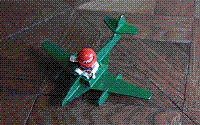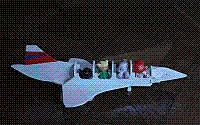## First Order IIR Filter and its implementation

The simplest IIR filter is a first order IIR filter. It attenuates signal above its cut-off frequency by 20dB/decade. How does the filter in figure 1 look in a code? Let's assume, that x is a result of analog to digital conversion which needs to be filtered. A new filter output is a sum of current ADC result multiplied by a and previous filter output multiplied by 1-a.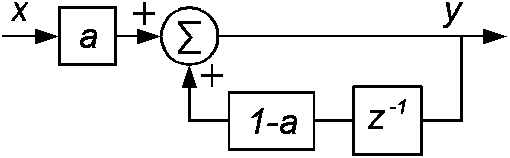Figure 1: The initial block diagram of first order IIR filter

As we can see in the figure 1, there are two variables x, y and two multiplications. When the input variable x is a byte, the output variable y must be a word to avoid rounding error. In the input path, the 8-bit long input variable x is multiplied by a constant a. This constant is very small. Usually, it is close to 0. When we want a low frequency attenuation to be 0dB, then a sum of both constants a and 1-a must be exactly one. So, the constant in feedback path 1-a is a little less than one. Because of the length of y, the multiplication in feedback path becomes cumbersome.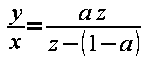(1)

The low frequency attenuation of 0dB is proved by equation 2. The equation is a filter's response to the unit step in infinity.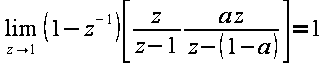(2)

To rearrange the block diagram, the constant in feedback is split to 1 and -a. First part of the feedback constant remains as is, but the second part -a is shifted to the sum point in front of input multiplication. As we can see in the figure 2, there is only one multiplication left.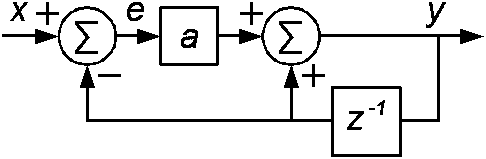Figure 2: The optimized block diagram of first order IIR filter

In the input sum point, a high byte of 16-bit long variable y is subtracted from the 8-bit long variable x to get a signed 8-bit long error e. When we choose the constant a to be 1/256, the multiplication shifts high byte of e to low byte of the product. The high byte of the product contains a sign which is extended from the low byte. So, the multiplication is converted to some byte manipulation. Finally, the word is added to the output integrating cell which is formed by the delay cell z-1 and sum point.

As we can see, it is possible to implement the first order IIR without any multiplications.

## Second order IIR Filter

The second order IIR filter attenuates signal above its cut-off frequency by 40dB/decade. Its transfer function is shown in equation 3.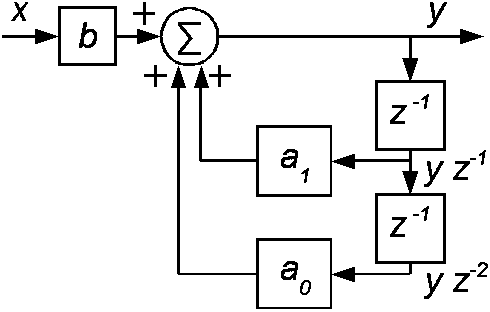Figure 3: The initial block diagram of second order IIR filter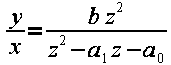(3)

To keep attenuation at low frequencies equal to 0dB, an equation 5 must be fulfilled.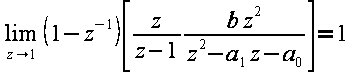(4)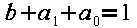(5)

Poles of transfer function 3 are chosen close to 1, as we can see in figure 4. The a sets filter's cut-off frequency. A damping of the filter is fixed to 0.7 to keep overshot close to zero.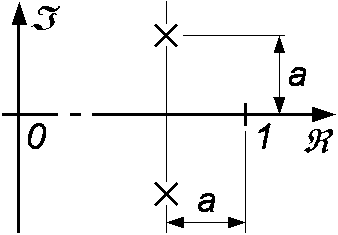Figure 4: Position of roots

When the attenuation and roots' position of filter are fixed, the equation 3 becomes equation 10.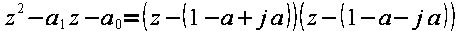(6)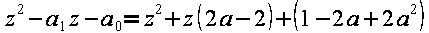(7)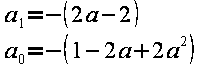(8)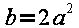(9)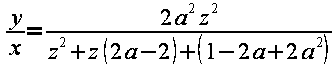(10)

The equation 10 is a common transfer function of second order IIR filter.

## Delaying of a filter

First, we are going to get rid of z2 from the nominator of equation 10. This is done by delaying the output by two sample periods. It depends on application, when this delay is acceptable.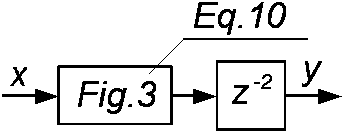Figure 5: The delayed second order IIR filter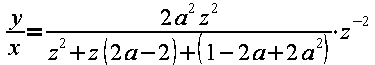(11)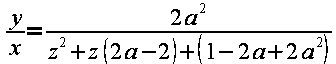(12)

The equation 12 is the transfer function of the delayed second order IIR filter and it is going to be a starting point in optimizing of the block diagram.

## Cascade of two first order IIR filters

To optimize the second order IIR filter, auxiliary calculations are needed to be carried out. First, the IIR filter from figure 2 is delayed for one sample period. The delay is a consequence of shifting of filter's output behind the delay block z-1 as we can see in figure 6. The equation 13 is a transfer function of the delayed filter.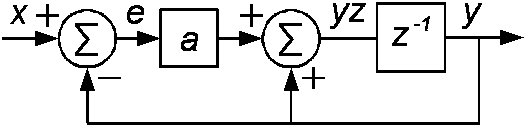Figure 6: The delayed first order IIR filter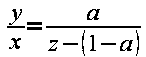(13)

Two of delayed first order IIR filters are then connected to a cascade. This means that output of first filter is input to the second one. The equation 14 is a transfer function of the cascade.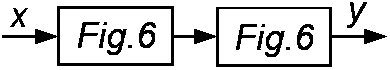Figure 7: The cascade of first order IIR filters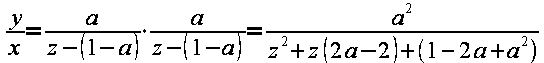(14)

When we can implement transfer function 1 in 8-bit microcontrollers easily, we could implement the transfer function 13 as well. When we double a code of transfer function 13, we obtain code for transfer function 14.

## Implementation of Second order IIR Filter

The transfer function 14 does not differ much from transfer function 12. This difference is going to be erased by a global feedback around the cascade that can be seen in figure 8. A block in the middle is the transfer function 14 which is written as a quotient in equation 15.

The multiplication in input path in figure 8 is a consequence of number 2 in the numerator of transfer function 12.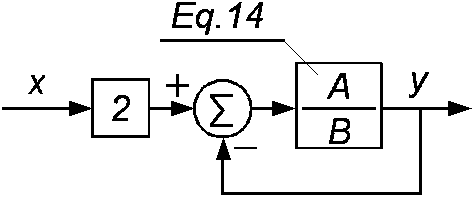Figure 8: The cascade with a global feedback loop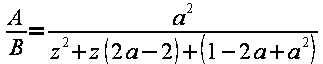(15)

The equation 16 is the transfer function of the block diagram in figure 8. We put in the numerator and denominator from equation 15 to get the transfer function 17 that we are looking for. It is the same as transfer function 12.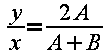(16)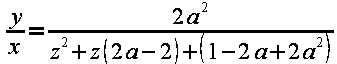(17)

The optimized block diagram of second order IIR filter is shown in the figure 9. There is a cascade of two delayed first order IIR filter from figure 6 which are surrounded by the global feedback. Of course, there is the multiplication by 2 in the input path.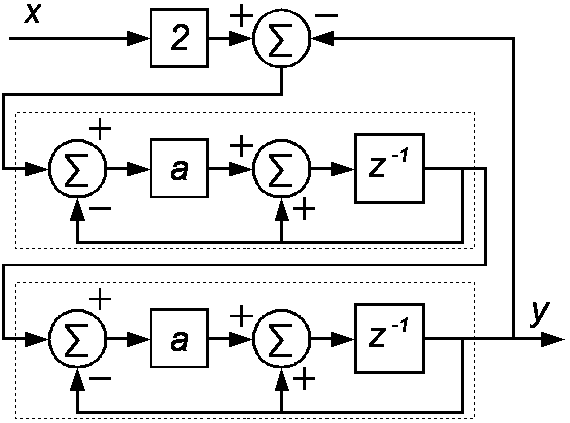Figure 9: The optimized block diagram of second order IIR filter

As it has been said in this article, the two first order IIR filters could be implemented without multiplication when a is selected to be 1/256. Then, a multiplication by 2 can be done by shifting. So, a second order IIR filter with damping of 0,7 can be coded without using any multiplication, only adding and byte manipulating. An 8-bit microcontroller is able to perform these two operations fast and easily. And, a second order IIR filter is no more a hard work for the 8-bit microcontroller.